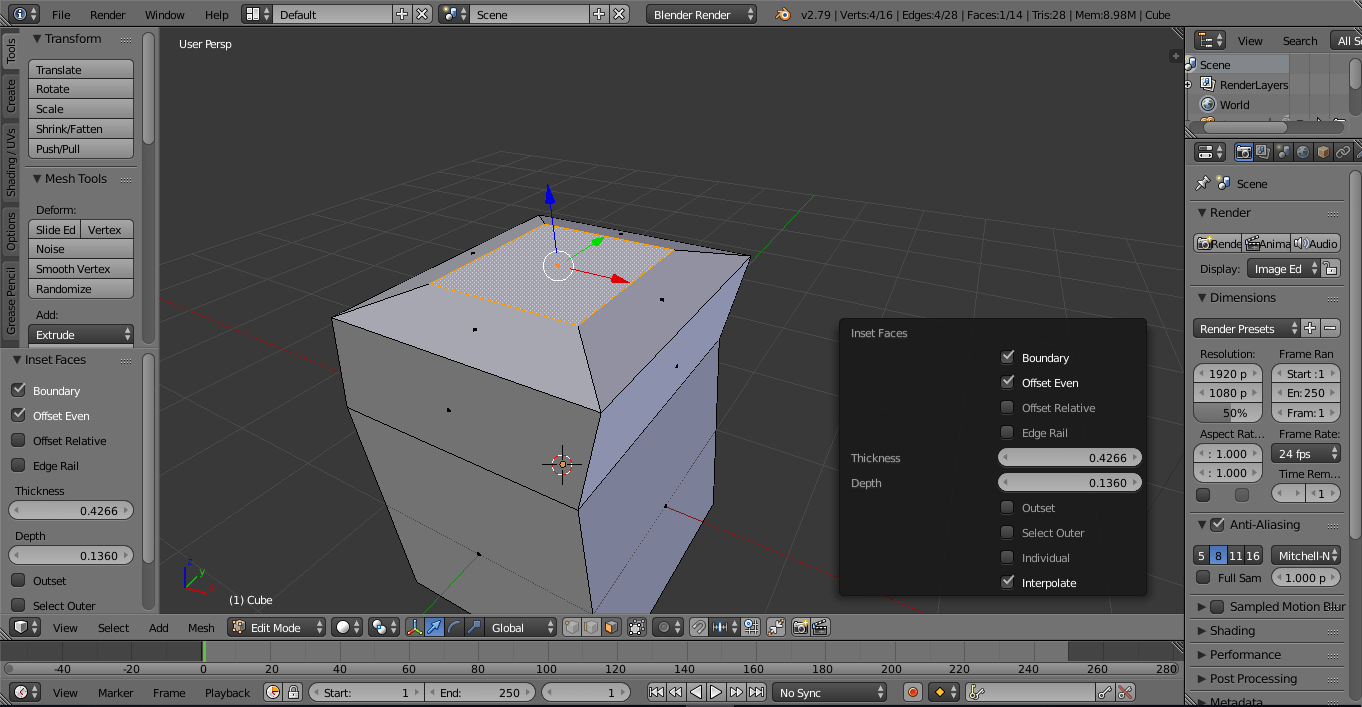## UE4 – Replace the Camera component icon

Software:
Unreal Engine 4.26

Note:
This seems like an awkward workaround..
So if I missed something here, and there’s a better method to do this,
I’ll be very grateful is you share it in the comments.
Also,
The following tip is only relevant for the CineCameraActor and won’t work with a regular CameraActor as it has a different built in offset (hopefully, I’ll have time to add this to the post later..)

Replacing the camera icon:
Its fairly simple to replace the Camera component’s mesh icon,
Just select the component and replace it’s Camera Mesh Static Mesh component with a different static mesh object: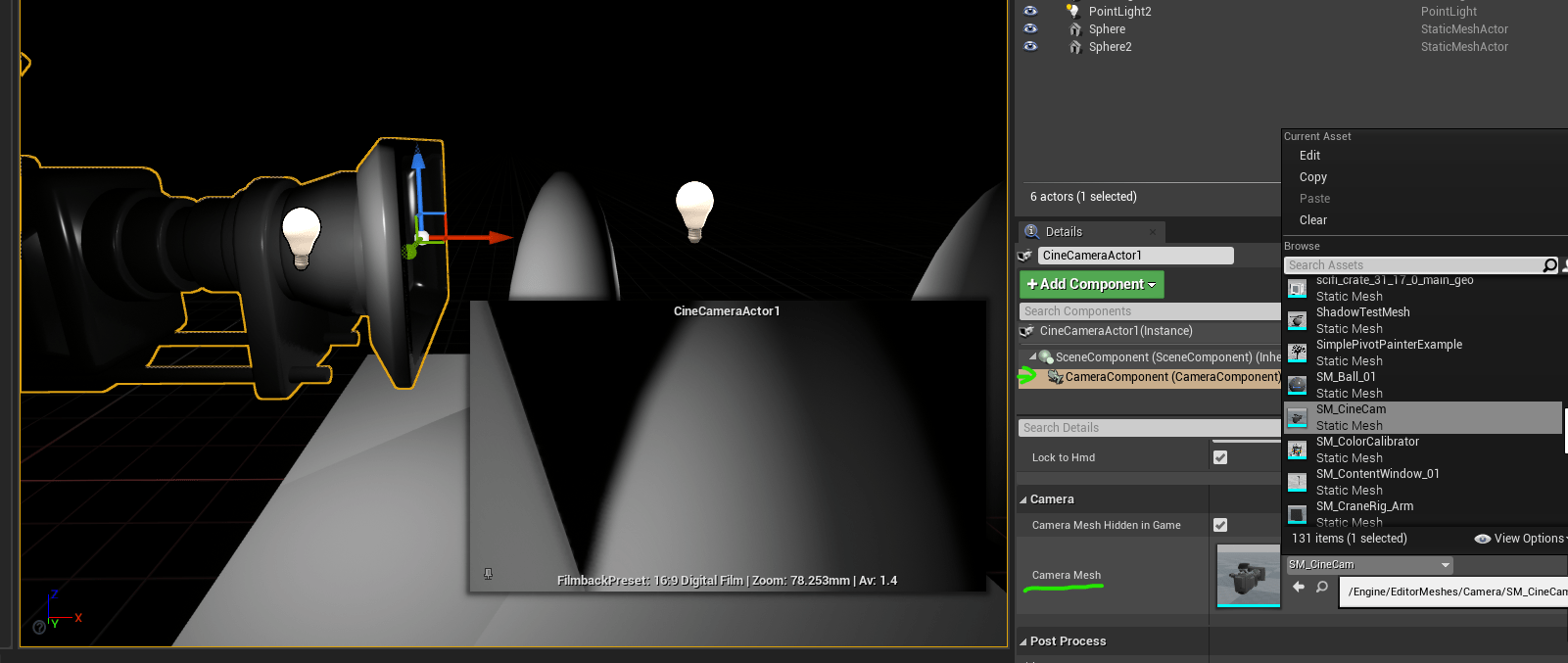So what’s the problem?
The problem is that the default mesh used for the camera icon doesn’t have its natural pivot at the focal point of the camera, but at its bottom somewhere,
And there is a hardcoded transform offset that compensates for that and places the icon mesh in a way that has the Icon lens roughly at the actual Camera actor pivot / focal point: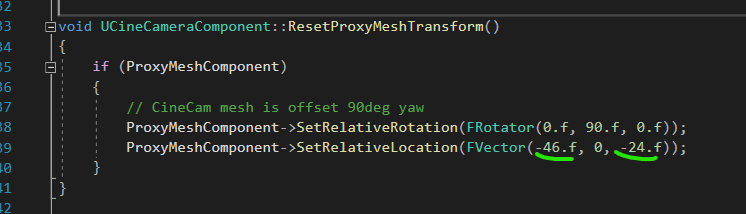* I haven’t found any exposed transform parameter that allows moving the icon itself without moving the camera.
So in-order to replace the camera mesh with an alternative icon mesh, and have it be aligned properly to the camera’s pivot / focal point (without changing engine code and building it) the built-in offset must be negatively pre-added to the new mesh model:In this example in Blender, a new icon is modeled facing positive Y, with pre-built offset to compensate for the hardcoded offset in UE.The Camera actor with the alternative Icon:Note:
In this example, I’ve replaced the camera icon with a much smaller model, intentionally, to suite a tiny scale project,
You can also scale the icon without replacing the model.

## Houdini – Separate continuous mesh parts to groups

Software:
Houdini 18.5

Separate a mesh to groups by continous parts (elements):

1. Use the Connectivity SOP node to assign each mesh primitive an integer attribute based on the continuous mesh element in belongs to.
This attribute can be named “element” or “part” for example.
2. Use the GameDev Group by Attribute* node to assign each mesh primitive to a different group according to its “part” attribute (if you named it “part” that is..).
Set a group name prefix, like “part_” or “element_”. the node will generate numbered groups per each mesh part like so: “element_0”, “element_1”, “element_1″….
3. Use this group separation as a filter to create whatever part-selective effect you need.

* The GameDev Group by Attribute node ships with the Game Development Toolset

## Blender Python – Reading mesh UV data

Software:
Blender 2.83

Simple example code for reading mesh UV data.

Link to this code snippet on gist

Note that there are typically many more mesh loops than vertices.
*Unless the case is a primitive undivided plane..

``````import bpy
access mesh data:
obj = bpy.context.active_object
mesh_data = obj.data
mesh_loops = mesh_data.loops
uv_index = 0
iterate teh mesh loops:
for lp in mesh_loops:
# access uv loop:
uv_loop = mesh_data.uv_layers[uv_index].data[lp.index]
uv_coords = uv_loop.uv
print('vert: {}, U: {}, V: {}'.format(lp.vertex_index,      uv_coords, uv_coords))``````

## Blender – Python – Access animated vertices data

Software:
Blender 2.83

The following is simple example of reading a mesh’s animated vertices data.

This example code gist

Note that accessing an model’s animated vertex locations requires reading the model’s evaluated (deformed) mesh state per frame.
For that reason a new bmesh object is initiated per each frame with the the model’s updated dependency graph.

``````import bpy
import bmesh
obj = bpy.context.active_object
frames = range(0,10)
get the object's evaluated dependency graph:
depgraph = bpy.context.evaluated_depsgraph_get()
iterate animation frames:
for f in frames:
bpy.context.scene.frame_set(f)
# define new bmesh object:
bm = bmesh.new()
bm.verts.ensure_lookup_table()
# read the evaluated mesh data into the bmesh   object:
bm.from_object( obj, depgraph )
# iterate the bmesh verts:
for i, v in enumerate(bm.verts):
print("frame: {}, vert: {}, location: {}".format(f, i, v.co))``````

## Blender Python – Accessing mesh triangles

Software:
Blender 2.81 | Python 3.74

By default, mesh triangles are not accessible to Python in Blender.
When accessing the mesh triangles is needed, they must be calculated first using the ‘calc_loop_triangles’ Mesh object method.
Before the calc_loop_triangle method has been called, the loop_triangles property of the Mesh object will reference a empty collection.
After calling the calc_loop_triangles method, the loop_triangles property will reference a collection of MeshLoopTriangle objects, in which the vertices property will hold an array of 3 integers that are the indices of the triangle vertices.

The following example script creates and places sphere objects at the centers of the cube’s triangles:

```import bpy
mesh = bpy.data.objects['Cube'].data
mesh.calc_loop_triangles()
for tri in mesh.loop_triangles:
tri_center = (mesh.vertices[tri.vertices].co * 0.333) +\
(mesh.vertices[tri.vertices].co * 0.333) +\
(mesh.vertices[tri.vertices].co * 0.333)
enter_editmode=False,
location=tri_center)```

* note that when copying and pasting a script from this example, the indentation may not be pasted correctly.

## Python for 3ds max – Mesh manipulation

Software:
3ds max 2019

An example of creating a mesh ripple deformation using Python for 3ds max: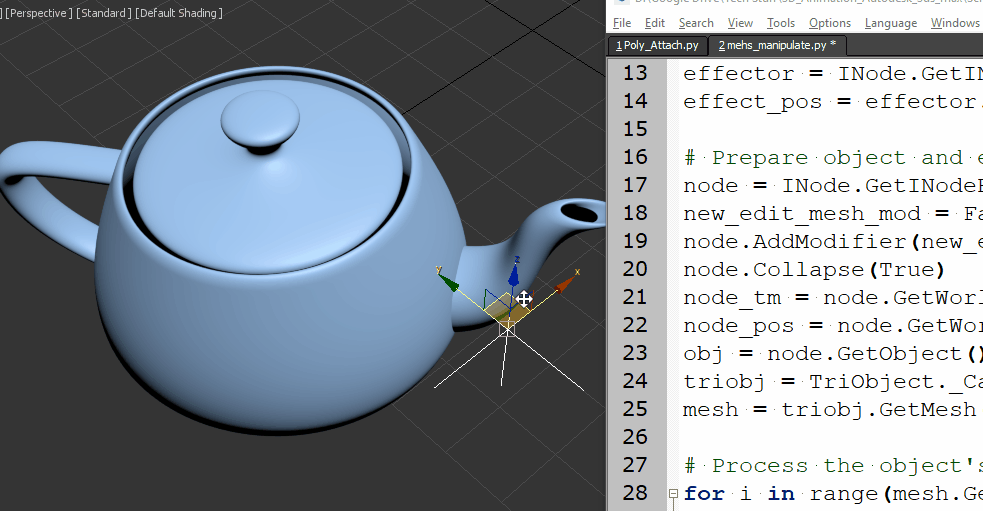Script steps:

1. Define the effect intensity and a helper point object that will serve as the effect center.
2. Collapse the object to an Editable-Mesh so its vertices will be accessible by Python.
Note that the Node‘s Object has to be cast as a TriObject, to access the object’s Mesh data.
3.  Loop through the Mesh’s vertices, get their world position, and set a new Z position as a sine function of the distance from the effect center.
```import math
from MaxPlus import Factory
from MaxPlus import ClassIds
from MaxPlus import INode
from MaxPlus import TriObject
from MaxPlus import Matrix3
from MaxPlus import Point3

# Intensity:
effecr_mult = 1.0

# Effect center:
effector = INode.GetINodeByName('Point001')
effect_pos = effector.GetWorldPosition()

# Prepare object and eccess it's mesh data:
node = INode.GetINodeByName('Teapot001')
new_edit_mesh_mod = Factory.CreateObjectModifier(ClassIds.Edit_Mesh)
node.Collapse(True)
node_tm = node.GetWorldTM()
node_pos = node.GetWorldPosition()
obj = node.GetObject()
triobj = TriObject._CastFrom(obj)
mesh = triobj.GetMesh()

# Process the object's vertices:
for i in range(mesh.GetNumVertices()):
# Get vertex in world space
vert_pos = mesh.GetVertex(i)
vert_world_pos = node_tm.VectorTransform(vert_pos)
vert_world_pos = vert_world_pos + node_pos
# Get vertex distance from effect center:
diff_vec = vert_world_pos - effect_pos
diff_vec.Z = 0
dist = diff_vec.GetLength()
# Set new vertex position:
mesh.SetVert(i,vert_pos.X,vert_pos.Y,vert_pos.Z + math.sin(dist)*effecr_mult)```

* note that when copying and pasting a script from this example, the indentation may not be pasted correctly.

Related Post:
Python for 3ds max – Animated Mesh

## Python for 3ds max – Animated Mesh

Software:
3ds max 2019

This is an example of procedurally animating a mesh’s vertices via Python script.Notes:
1. The model has to be converted to Editable Mesh before the script is run.
* unless the scrip will be extended to do it.
2. The model must be selected for the script to work.

```import MaxPlus
import math
from MaxPlus import INode
from MaxPlus import TriObject
from MaxPlus import SelectionManager
from MaxPlus import Factory
from MaxPlus import Animation
from MaxPlus import Point3
from MaxPlus import Control

ticks_frame = 160

#Selection
sel = []
for n in SelectionManager.Nodes:
sel.append(n)
node = sel

#Setup Controllers
obj = node.GetObject()
Tri = TriObject._CastFrom(obj)
mesh = Tri.GetMesh()
num_verts = mesh.GetNumVertices()
mesh_anim = obj.GetSubAnim(0)
pnt_ctrl = Factory.CreateDefaultMasterPointController()
node.AssignController(pnt_ctrl,1)
for i in range(num_verts):
bezp3 = Factory.CreateDefaultPoint3Controller()
bezp3.SetPoint3Value(mesh.GetVertex(i))
mesh_anim.AssignController(bezp3,i)

#Animation
Animation.SetAnimateButtonState(True)
for t in range(100):
time = t * ticks_frame
Animation.SetTime(time)
for i in range(num_verts):
vert_anim = mesh_anim.GetSubAnim(i)
vert_ctrl = Control._CastFrom(vert_anim)
vert_val = mesh.GetVertex(i)
vert_val.SetZ(vert_val.GetZ() + math.sin(((Animation.GetTime()*0.5)/(ticks_frame))+i))
vert_ctrl.SetPoint3Value(vert_val)
Animation.SetAnimateButtonState(False)```

* note that when copying and pasting a script from this example, the indentation may not be pasted correctly.

## Blender – Turn deformations and shape keys to mesh

Software:
Blender 2.79

To apply shape key or modifier deformations to the mesh:

Either:

1. Press Ctrl + A to open the Apply commands menu.
2. Choose Apply Visual Geometry to Mesh.

Or:

1. Press Alt + C.
2. Choose Mesh From Curve/Meta/Surf/Text.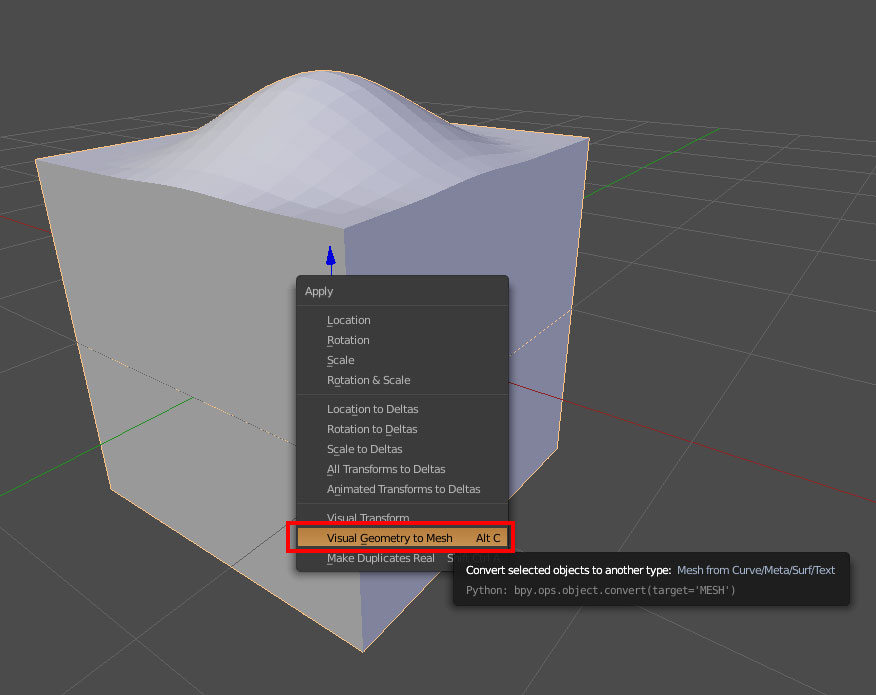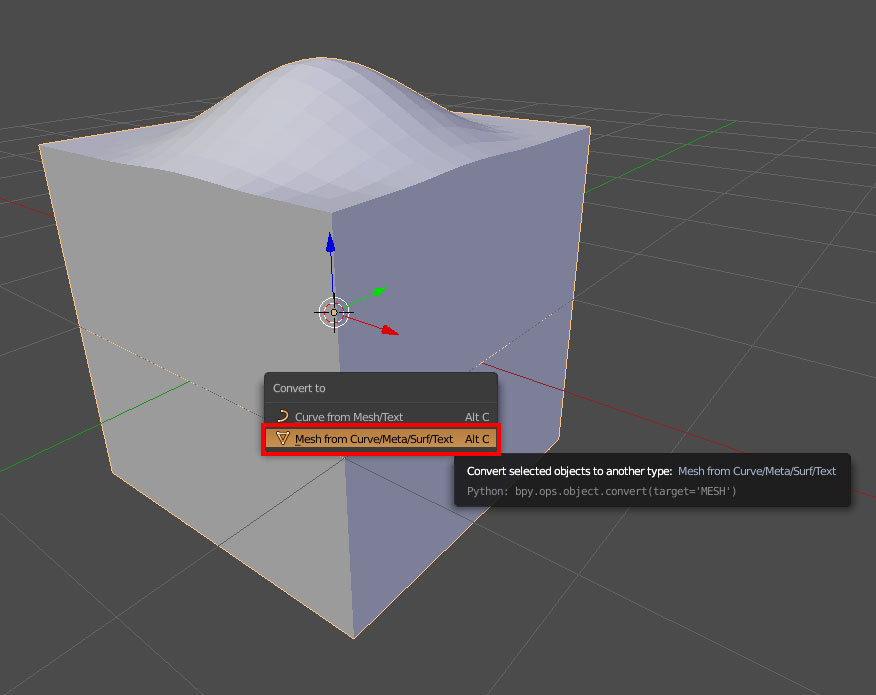## Convert particles to mesh in Blender

Software:
Blender 2.82

1. Select the particle emitter object.
2. In the particle system modifier, press the Convert button or press Ctrl Alt A.

Note:
The objects that will be created will all have their mesh data linked to the original Dupli-Object (they will be instances of the particle object chosen in the particle system Render Settings)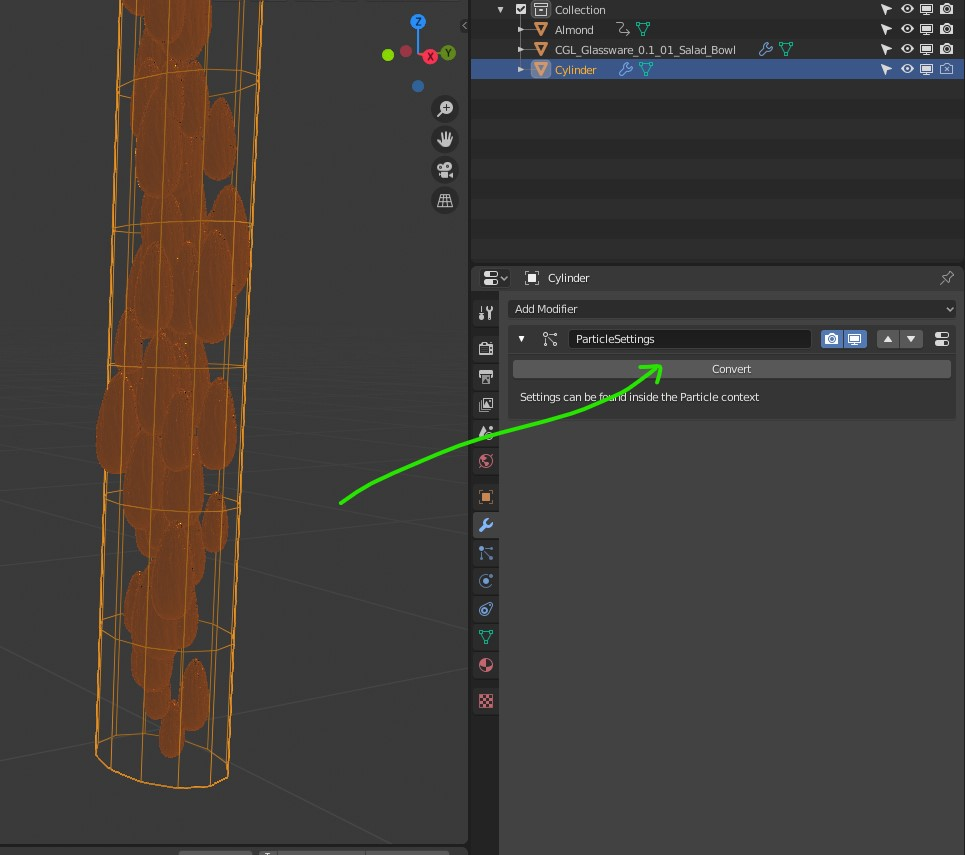## Shortcut to mesh editing tool settings in Blender

Software:
Blender 2 .79

When editing mesh,
Press F6 to bring up the tool settings in a floating window: